# D105: Connecting the pressure sensor

### Pressure sensor for water, gas, air  for G1/4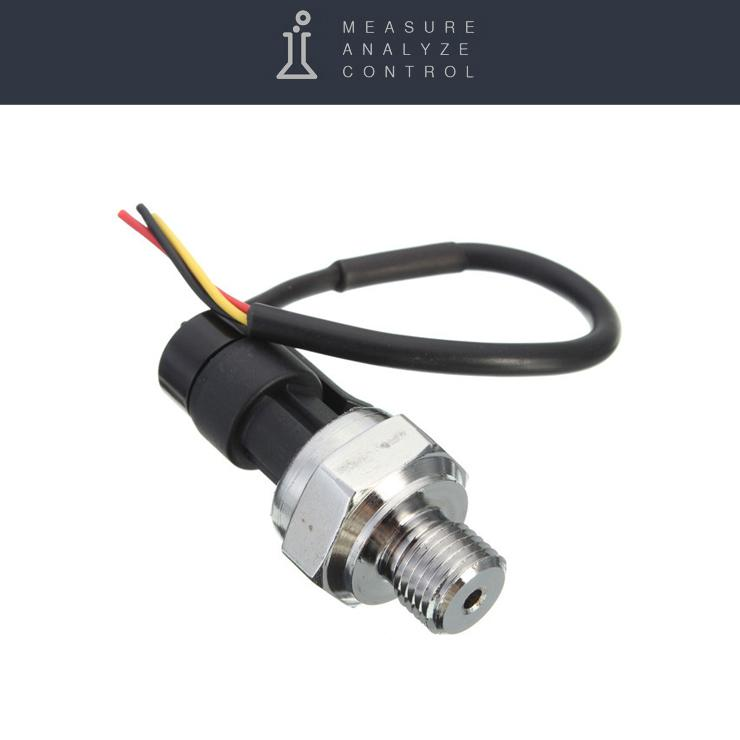A sensor with standard 1/4 '' plumbing thread can be connected to the smart-MAC D105 universal counter for measuring water or air pressure up to 1.2 MPa / 12 bar / 175 psi.

Wiring diagram:

• connect the black wire of the sensor to terminal 1: GND (Ground or -5V)
• connect the red wire of the sensor to terminal 6: + 5V
• connect the yellow sensor wire to terminal 5: ADC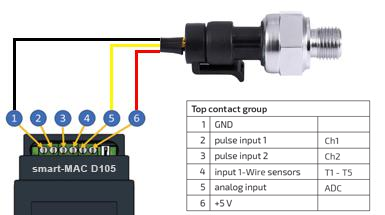Connection diagram pressure sensor to smart-MAC D105

#### Dashboard widget settings

Add a widget indicator, chart or table to the board.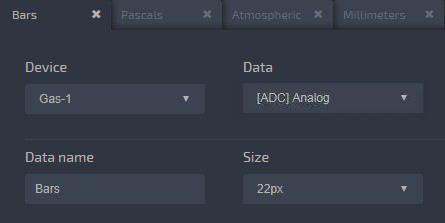In the section "Arithmetic operation" select the function f (x):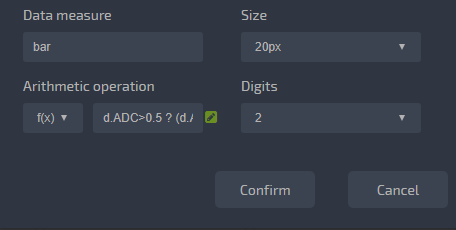At the output of the sensor, the voltage varies between 0.5 - 4.5V for pressure in the range 0 - 12 bar.

Accordingly, the voltage at the output of the sensor varies within 4V, and at 1V there are 3 bars.

In the section "Arithmetic operation" select the function f (x) and write down the function for calculating the pressure in the units you need:

Bars [bar]

`d.ADC > 0.5 ? (d.ADC-0.5)*3 : 0`

Pascals [MPa]

`d.ADC > 0.5 ? (d.ADC-0.5)*3*0.1 : 0`

Atmospheres [atm]

`d.ADC > 0.5 ? (d.ADC-0.5)*3*0.986923 : 0`

Millimeters of mercury [mm Hg Art.]

`d.ADC > 0.5 ? (d.ADC-0.5)*3*750.06 : 0`

Pound per square inch [psi]

`d.ADC > 0.5 ? (d.ADCx-0.5)*3*14.5038 : 0`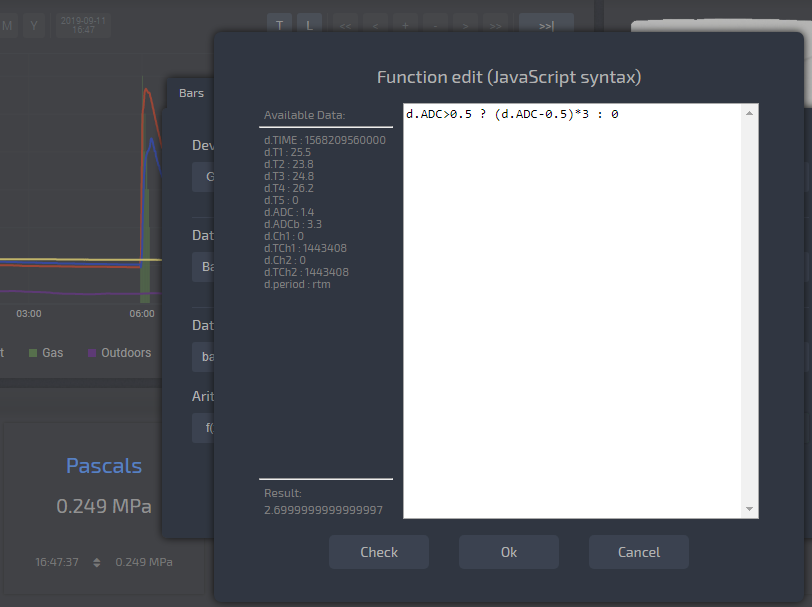#### Pressure sensor calibration.

Normally, the pressure sensor does not need to be calibrated. But if you have also a control pressure gauge and you really want the pressure on the sensor to match your pressure gauge, then you can build a more accurate linear relationship equation for two control points.

Record the voltage at the ADC input at low pressure and at high pressure. Knowing the value of pressure - voltage at two control points, use the halyard to build a linear relationship equation.

Pressure - sensor calibration.xlsx

#### Set up notifications on the device and control the built-in relay.

To configure notifications on the D105 device and automatically control the built-in relay according to the pressure level, it is necessary to determine the formula for calculating the voltage at the analog input at the corresponding pressure level.

`Vout = ( BAR + 1.5) / 3`

Where:
BAR - the pressure level in bars, at which an alert should come or a relay is activated;
Vout - the voltage at the analog input, at the appropriate pressure.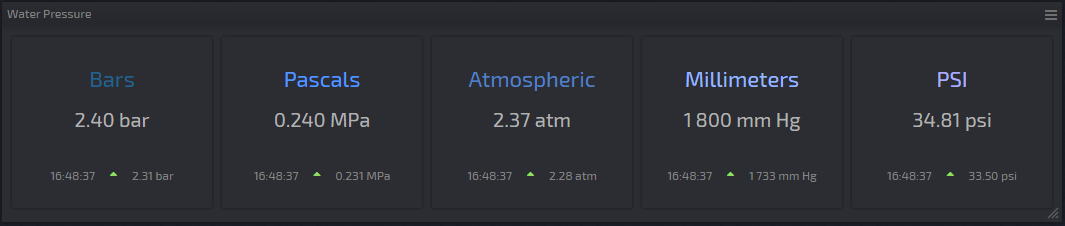Done!